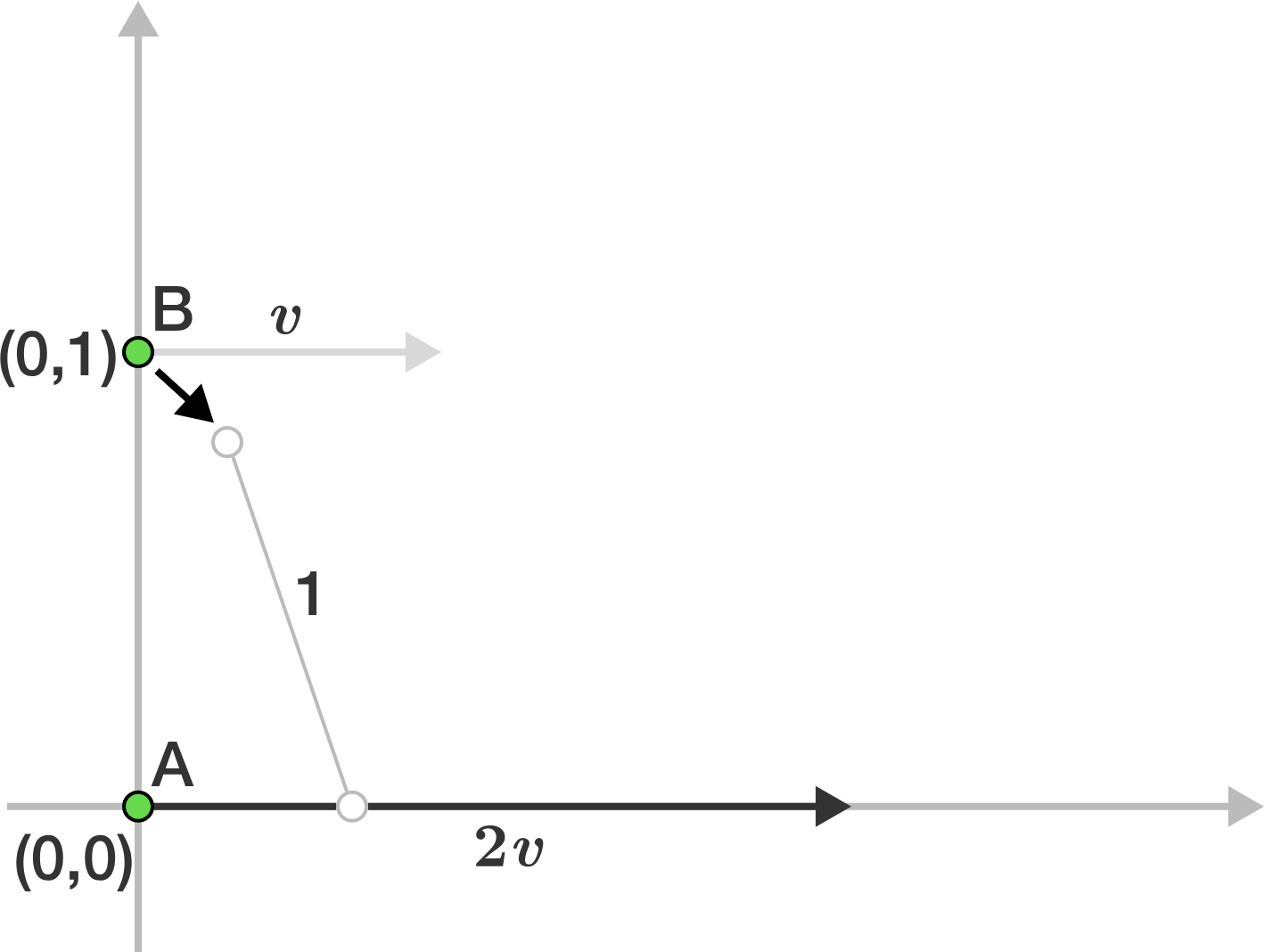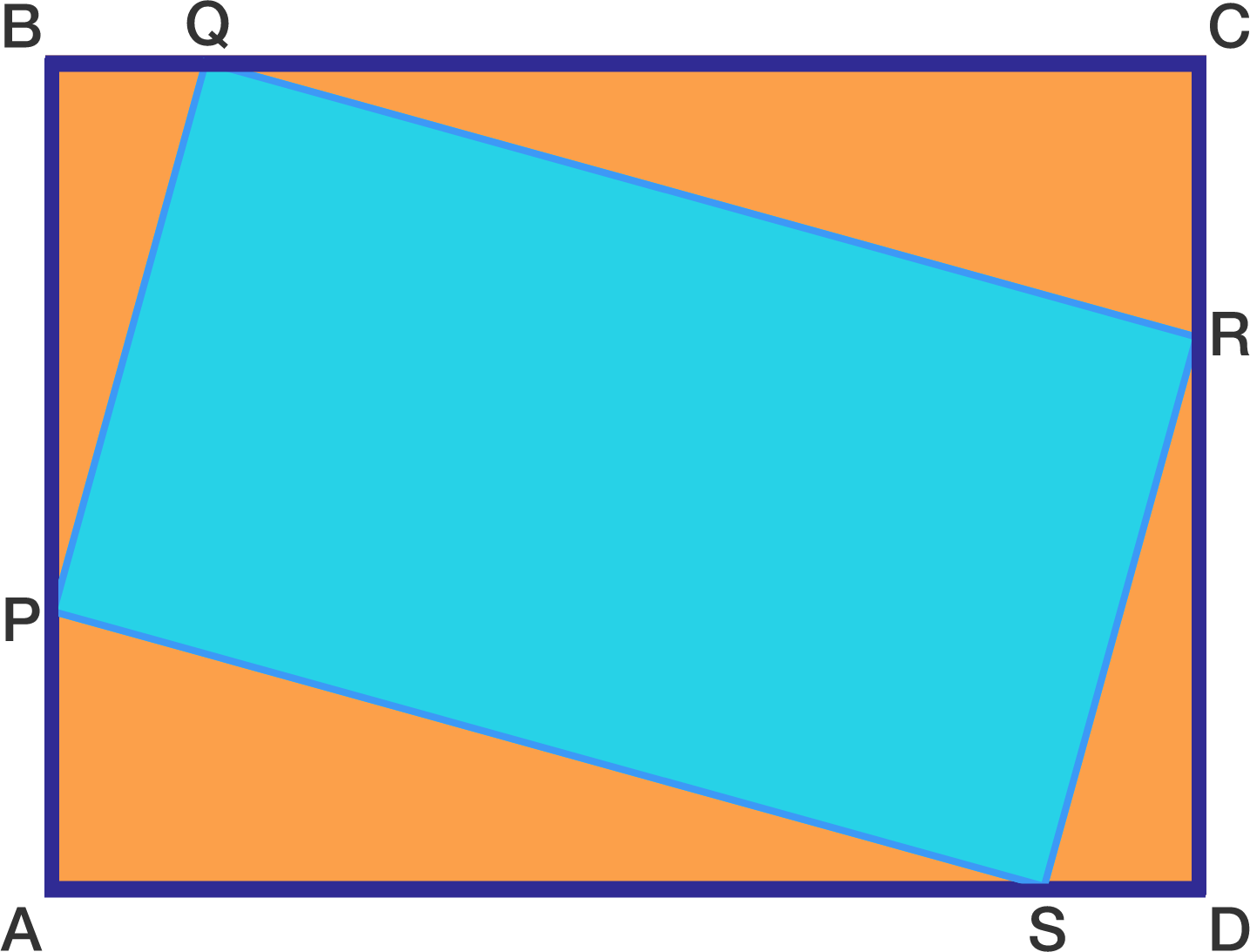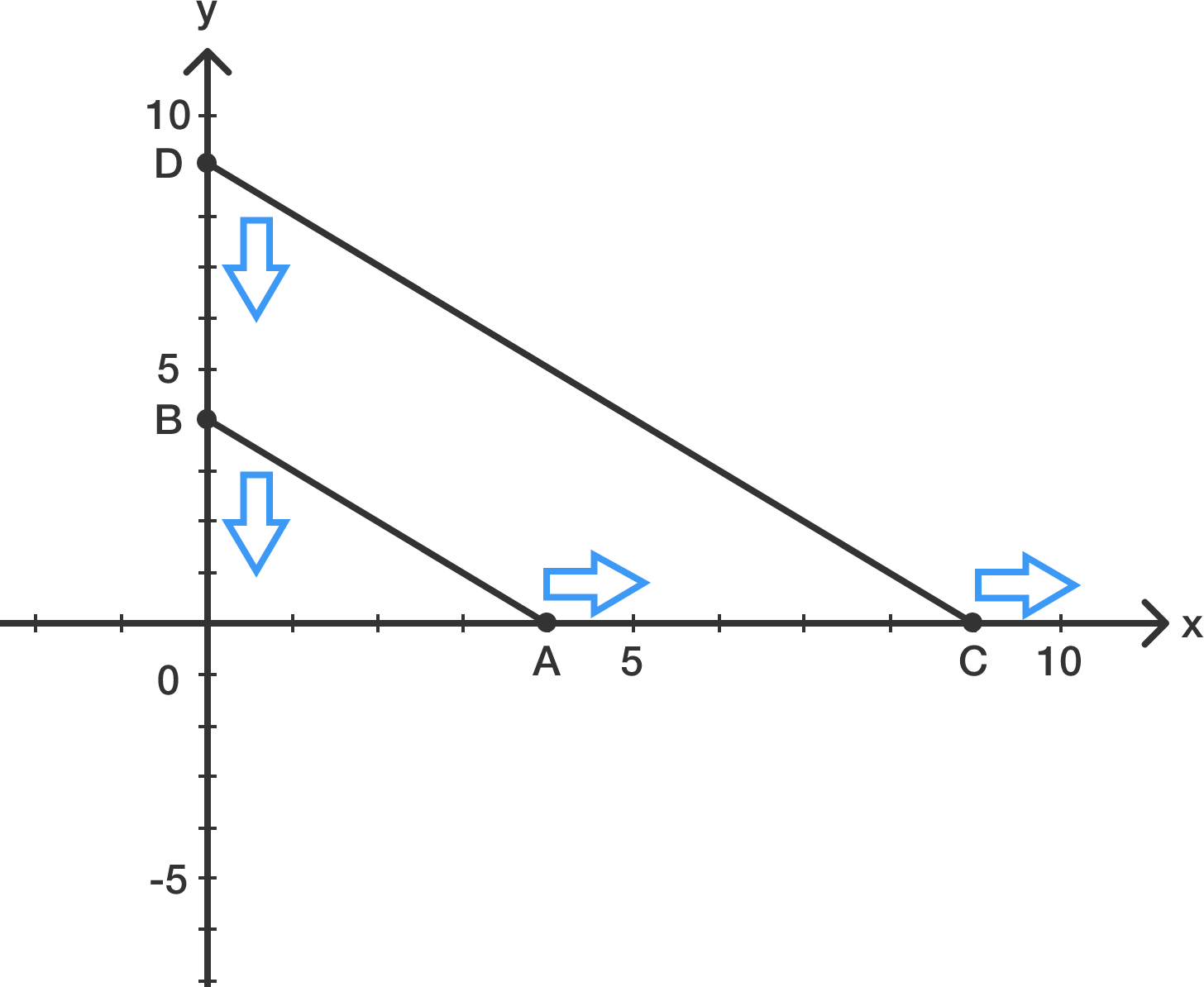Geometry

# 2D Coordinate Geometry: Level 5 ChallengesThere exists $2$ points on Cartesian coordinate. Point $B$ has coordinates $(0,1)$. Point $A$ is on the origin $(0,0)$.

Point $A$ and $B$ always has a constant distance of $1$ from each other.

Point $B$ moves with a horizontal velocity (parallel to the x-axis) of $v.$

Point $A$ moves with a horizontal velocity of $2v.$

Point $A$'s vertical velocity (parallel to the y-axis) is $0$ while Point $B$ is allowed to move vertically in order to keep the constant distance of $1$.

All this movement is happening in the first quadrant.

Let $P$ be the area made by the figure defined by: the $x$ axis, the $y$ axis, and the path traveled by point $B$ up to where it meets the $x$ axis. Find $\left\lfloor 1000P \right\rfloor .$

After you solve this, you might want to try a continuation of this problem.

Try my Other ProblemsIn the diagram above, ABCD and PQRS are both rectangles. Points P, Q, R, and S lie on segments $\overline{AB}$, $\overline{BC}$, $\overline{CD}$, and $\overline{DA}$, respectively, and $\overline{BQ} < \overline{QC}$.

If $AB=36$ and $BC=50$, then the maximum possible value of $BQ$ can be written in the form $a-\sqrt{b}$, where $a,b \in \mathbb{N}$. What is $a+b$?

Given line $x+2y=3$ intersects circle $x^2+y^2+x-6y+a=0$ at $P$ and $Q$. If $O$ is the origin and $OP\perp OQ$, what is the value of $a$?Let two Rods $AB$ of length $10$ and $CD$ of length $20$ are sliding on smooth standard co-ordinate axis such that their ends are always con-cyclic. If the locus of centre of that circle which pass through all four points $A,B,C,D$ is expressed as:

$(ax-by)^2 + (bx-ay)^2 = c^2$

For positive integers $a,b,c$. What is the minimum value of $a+b+c$

Details and Assumptions

• Diagram not up to scale
###### Inspired from Kushal Patankar's Problem

Two lines pass through the point $(-6, 7)$ and each is a distance of 2 from the origin at their closest to the origin. What is the sum of the slopes of these lines?

×